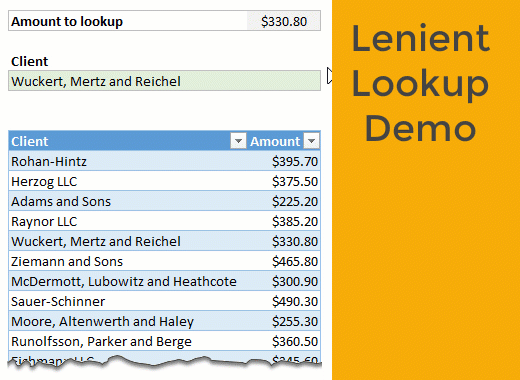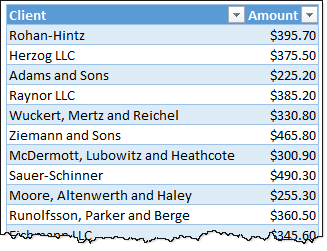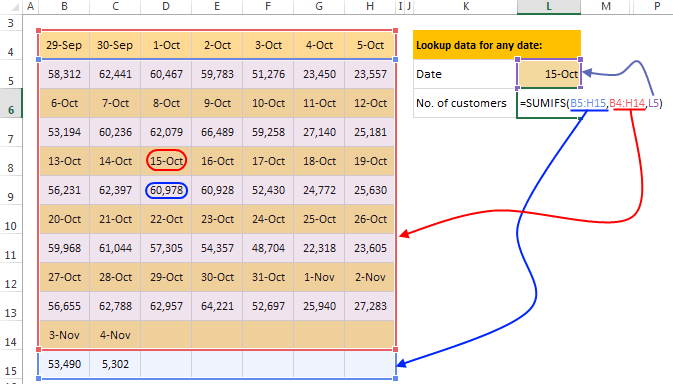Lenient lookup [Advanced Formula Trick] - KING OF EXCELKINGEXCEL.INFO ( KING OF EXCEL ) Welcome KINGEXCEL.INFO - Nothing Is Unable ... About Excel Tricks, Learning VBA Programming, Dedicated Software, Accounting, Living Skills ...

# Lenient lookup [Advanced Formula Trick]

We all know VLOOKUP (or INDEX+MATCH) as an indispensable tool in our Excel toolbox. But what if you want the lookups to be a little gentler, nicer and relaxed?
Let’s say you want to lookup the amount \$330.50 against a list of payments. There is no exact match, but if we look 50 cents in either direction, then we can find a match. Here is a demo of what I mean.Unfortunately, you can’t convince VLOOKUP to act nice.
Hey VLOOKUP, I know you are awesome and all, but can you cut me some slack here?
VLOOKUP is tough, reliable and has a cold heart. Or is it?
In this post, let’s learn how to do lenient lookups.

### Data for the problem

Let’s say you have a simple 2 column table like this. Our table is uninspiringly named data.## Lenient lookup – setting up the formula

Our input amount is in cell C3.
Let’s say when looking up for the amount, we want to follow this logic.
1. If an exact match is found, return that
2. Else, see if we can find anything with in 50 cents either side (you can change 50 to whatever you want)
3. If nothing can be found, we want to return “Not found” or similar message
Formulas to use:
1: we can use good old INDEX+MATCH
2: we can use array based INDEX+MATCH
3: we can use IFERROR.
Let’s put everything together.
Our lenient lookup formula (array):
`=IFERROR( INDEX(data[Client], IFERROR(MATCH(\$C\$3,data[Amount],0), MATCH(1, (data[Amount]>(\$C\$3-0.5))*(data[Amount]<(\$C\$3+0.5)),0) )),"Not found")`
How does it work?
Let’s go inside out.
MATCH(\$C\$3,data[Amount],0): this formula simply looks for C3 in data[Amount] column and returns the position.
MATCH(1, (data[Amount]>(\$C\$3-0.5))*(data[Amount]<(\$C\$3+0.5)),0): This array formula checks for 1 (TRUE) by looking at data[Amount] between C3-0.5 and C3+0.5
The formula has two Boolean arrays multiplied and it returns a bunch of 1s & 0s.
MATCH then picks up the first such amount.
Inner IFERROR(MATCH(…), MATCH(…)): This acts like a fail-safe switch. If there is no exact match (first one), then lenient match (second one) will be used.
Outer IFERROR(): If no matches are found (exact or lenient) then “Not found” will be printed.
As this is an array formula, you need to press CTRL+Shift+Enter to get the result.

## Other lenient / almost lookup problems

There are few more variations to this technique. Let’s review them.
Note: all of these are array formulas, so press CTRL+Shift+Enter.

### Ignore decimal portion

We lookup just the whole number portion of the value to find match.
Formula: =INDEX(data[Client], MATCH(G7, INT(data[Amount]),0))
Notes on how it works:
• INT() turns data[Amount] column to whole numbers.
• We then lookup the amount (G7) and return the match

### Amount is at least something, client name begins with S

Formula: =INDEX(data[Client], MATCH(1, (data[Amount]>=G8)*(LEFT(data[Client],1)=”S”),0))
• We use a different Boolean structure with >= and LEFT() formulas. The output will be a bunch of 1s & 0s.
• INDEX+MATCH for find the first such value (G8)

### Closest Amount to input

This is interesting. We use MIN & ABS to find closest amount to input value (G10) and return the client’s name.
Formula: =INDEX(data[Client], MATCH(MIN(ABS(data[Amount]-G10)), ABS(data[Amount]-G10),0))
• ABS(data[Amount]-G10) gives a bunch of absolute (positive) values. The smallest of these will closest to G10.
• MIN() finds the smallest value
• MATCH looks up the minimum value from ABS(data[Amount]-G10)
• INDEX gives corresponding client’s nameLookups are an essential part of any data analysis work you do in Excel. Pick up some nifty tricks from these links.